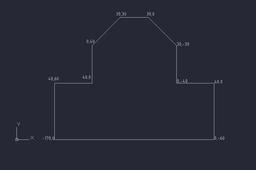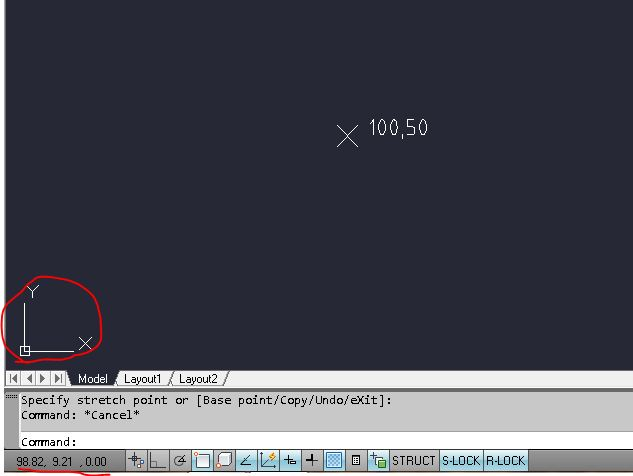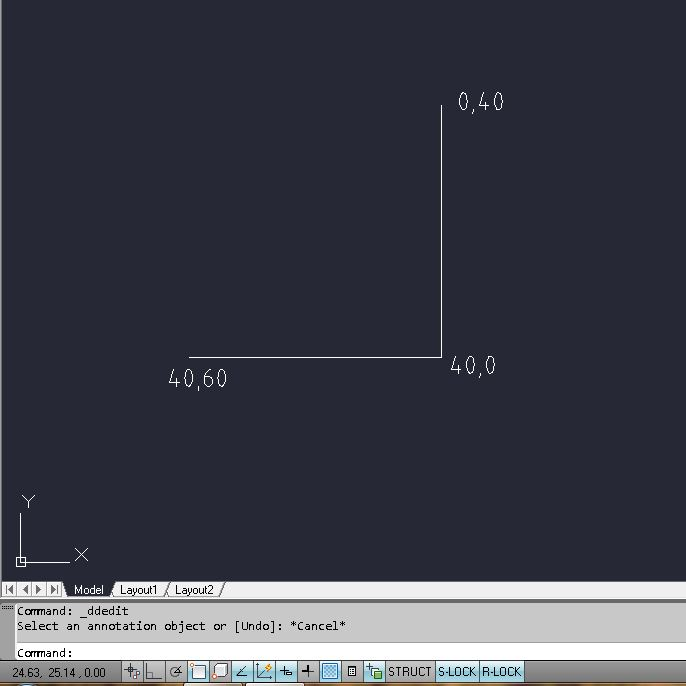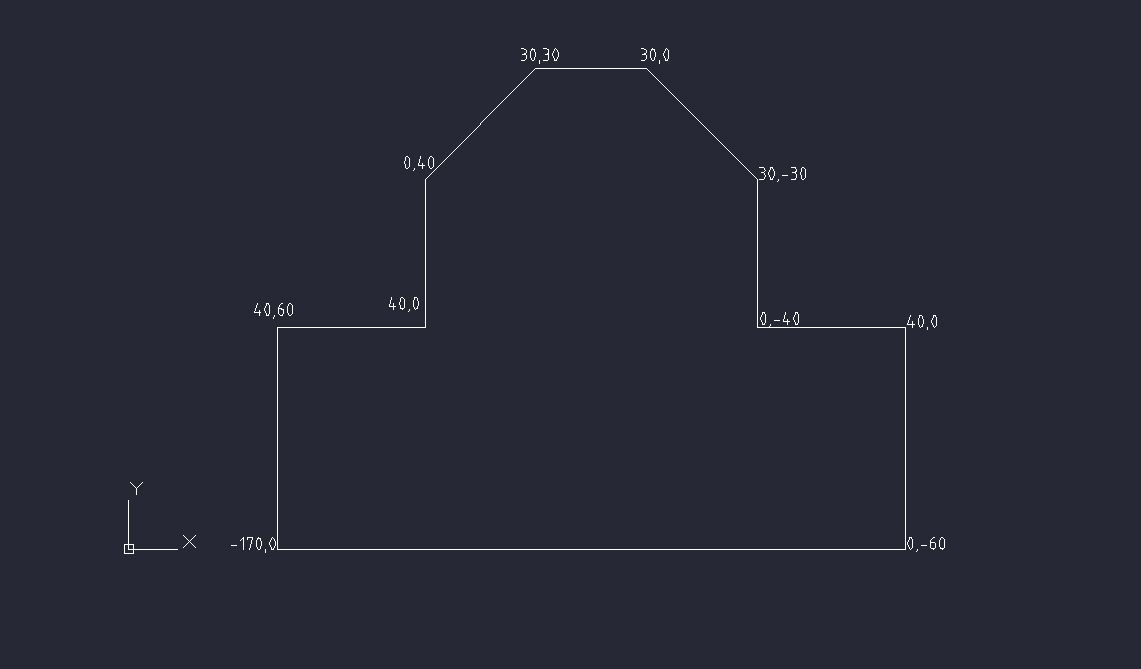# Drawing with coordinates in AutoCADIn this tutorial I am going to show you how to create drawings by using coordinates.

1. ### Step 1:To draw something by using coordinates you must know how UCS works.

That is the icon at the bottom left corner as you probably know.

You can see on the picture that we have a point which position is 100 mm from the UCS

on the X axis and 50 mm on the Y axis.

2. ### Step 2:On this picture we can see two perpendicular lines which have 3 coordinates.

The first coordinate (40,60) is relative to your UCS.

Just select the line command and type: 40,60 and it will automatically

start the first point of the line.

If you have problem with zooming in or out use REGEN command.

The second point of your line will be in (40,0) .

That is because your second point is relative to your first point so it is

40 in the X direction and 0 in the Y direction so the line will be horizontal.

The third point (0,40) follows the same process but this time it is

0 in the X direction and 40 in the Y direction so the line will be vertical

and perpendicular to the first one.

3. ### Step 3:Here, try to draw something like this, just do not forget how coordinate system works :)